# Minimum Fluidizing Velocities for Various Bed Packings By

• Slides: 30Minimum Fluidizing Velocities for Various Bed Packings By Andrew Maycock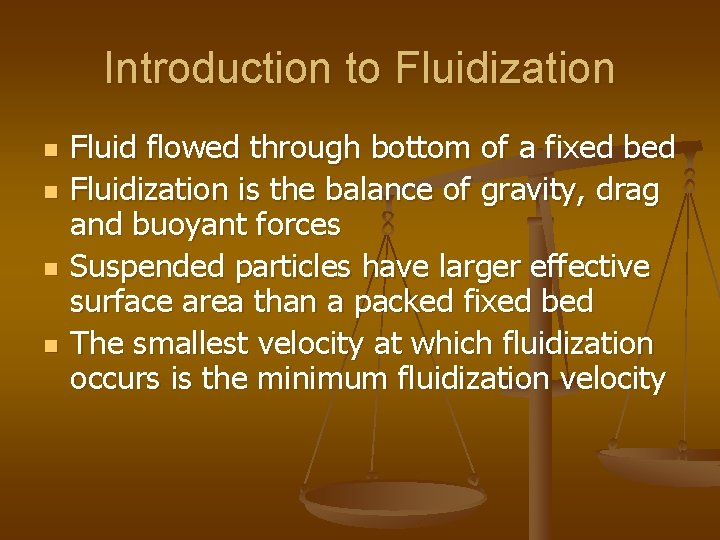Introduction to Fluidization n n Fluid flowed through bottom of a fixed bed Fluidization is the balance of gravity, drag and buoyant forces Suspended particles have larger effective surface area than a packed fixed bed The smallest velocity at which fluidization occurs is the minimum fluidization velocity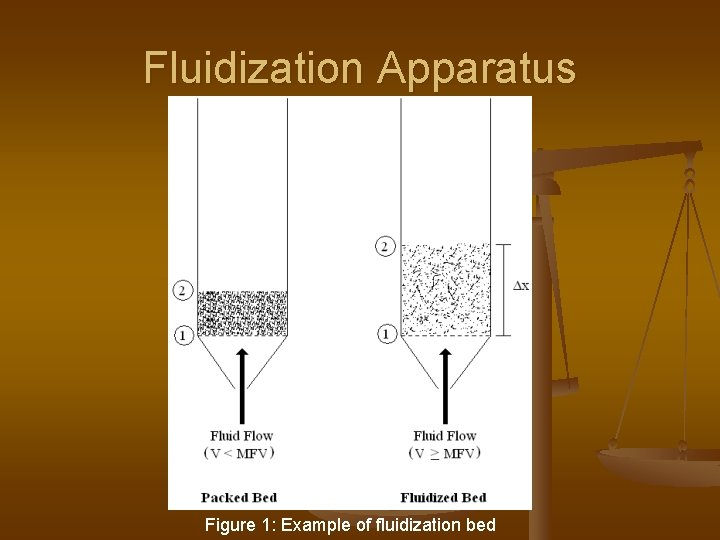Fluidization Apparatus Figure 1: Example of fluidization bedOverview n n n Theoretical Approach Experimental Approach Results Method Summaries Conclusions Q&A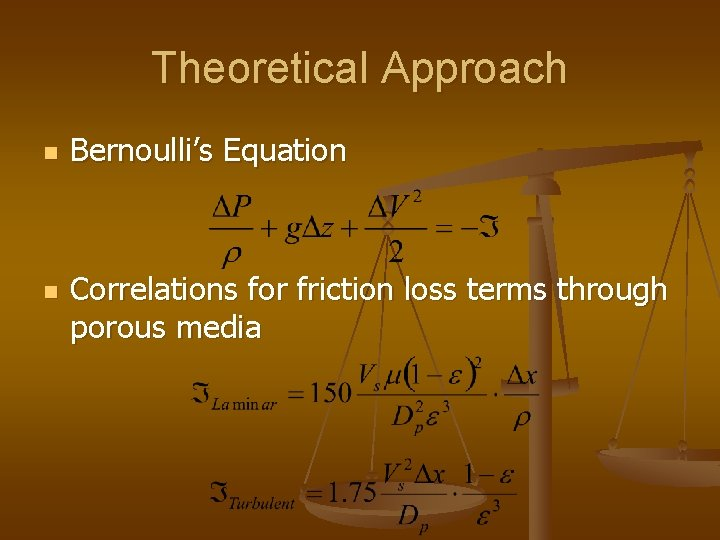Theoretical Approach n n Bernoulli’s Equation Correlations for friction loss terms through porous media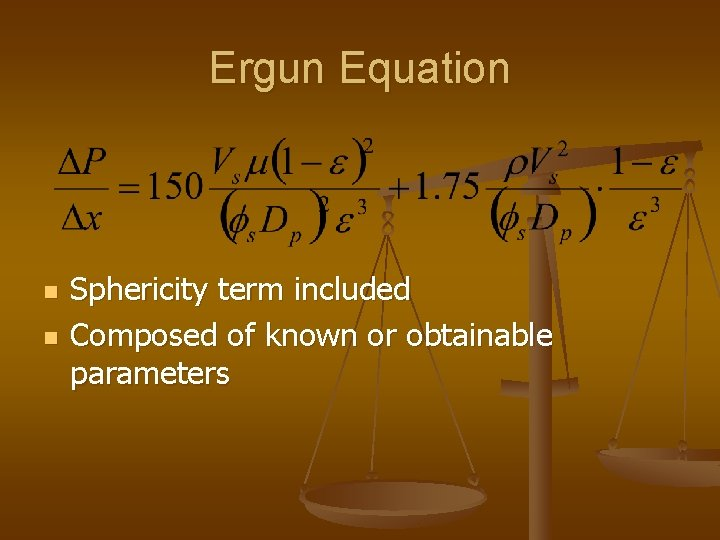Ergun Equation n n Sphericity term included Composed of known or obtainable parameters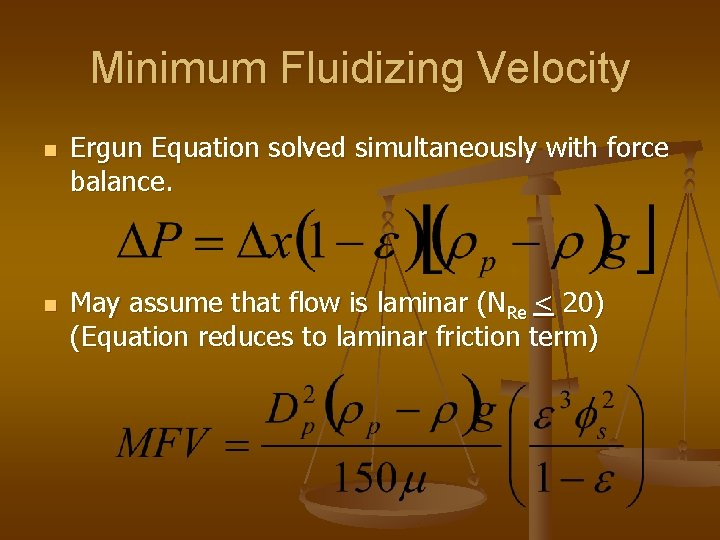Minimum Fluidizing Velocity n n Ergun Equation solved simultaneously with force balance. May assume that flow is laminar (NRe < 20) (Equation reduces to laminar friction term)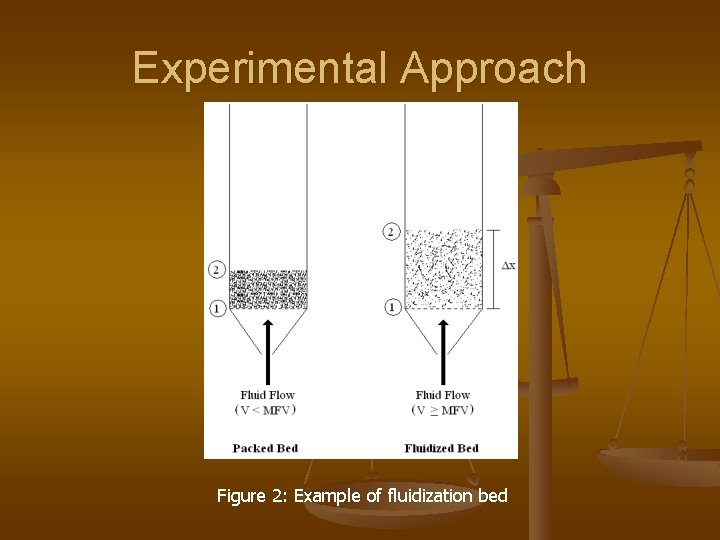Experimental Approach Figure 2: Example of fluidization bed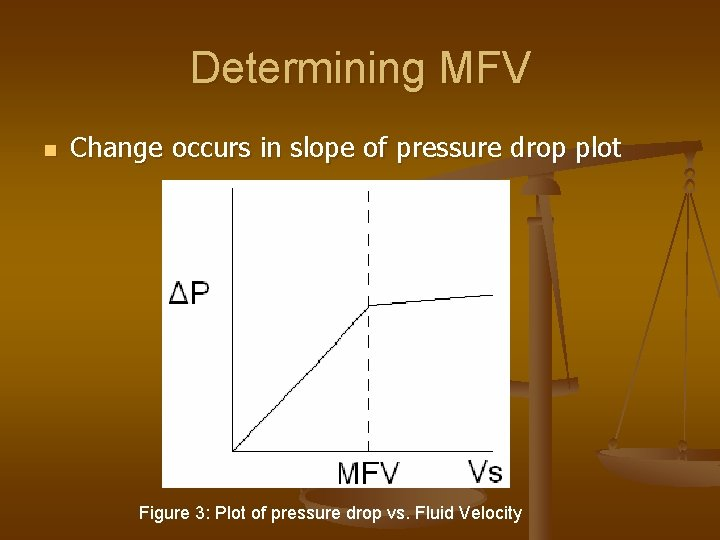Determining MFV n Change occurs in slope of pressure drop plot Figure 3: Plot of pressure drop vs. Fluid Velocity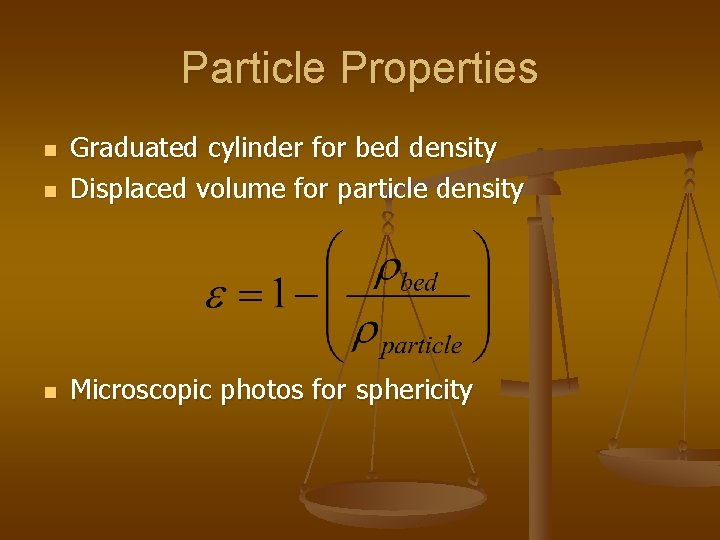Particle Properties n Graduated cylinder for bed density Displaced volume for particle density n Microscopic photos for sphericity n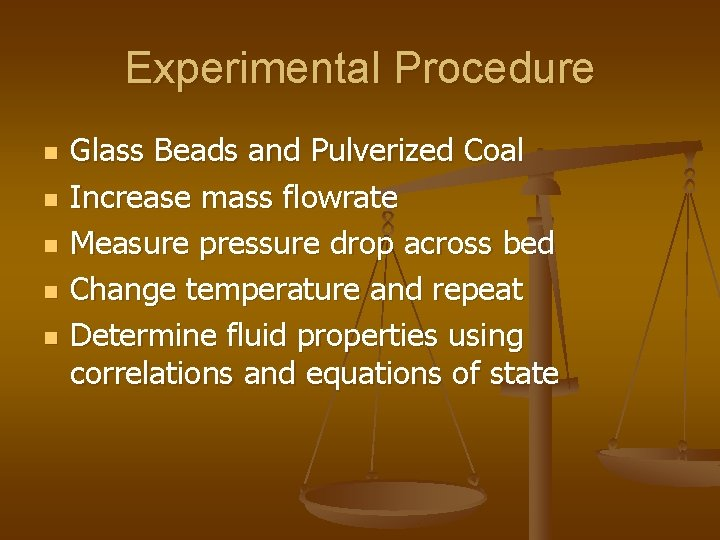Experimental Procedure n n n Glass Beads and Pulverized Coal Increase mass flowrate Measure pressure drop across bed Change temperature and repeat Determine fluid properties using correlations and equations of state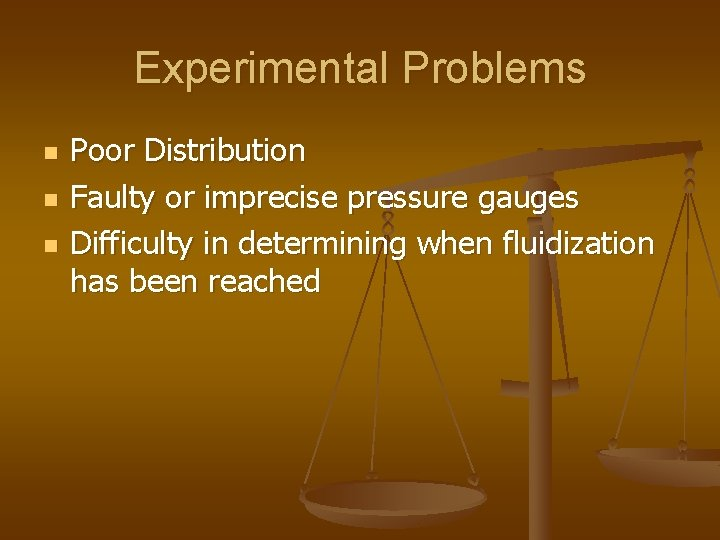Experimental Problems n n n Poor Distribution Faulty or imprecise pressure gauges Difficulty in determining when fluidization has been reachedResultsPulverized Coal Results Figure 3: Microscopic photo of pulverized coal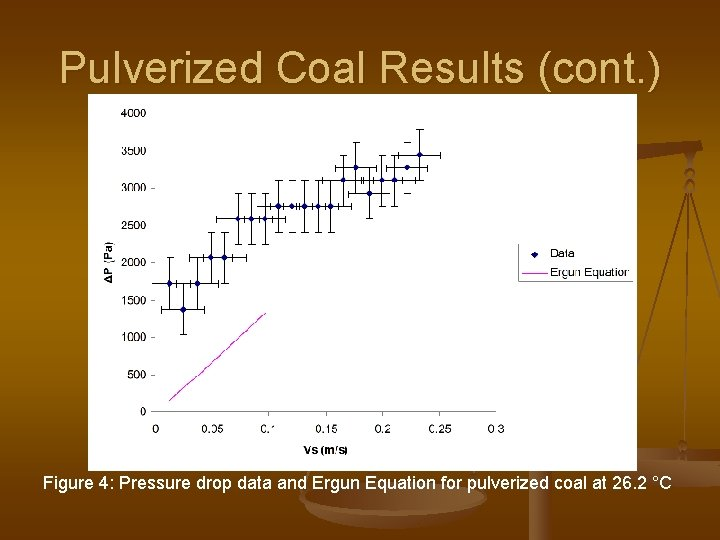Pulverized Coal Results (cont. ) Figure 4: Pressure drop data and Ergun Equation for pulverized coal at 26. 2 °C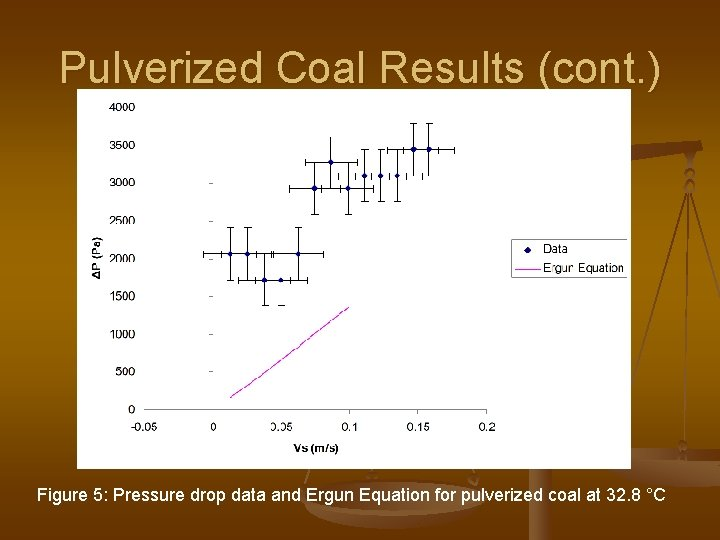Pulverized Coal Results (cont. ) Figure 5: Pressure drop data and Ergun Equation for pulverized coal at 32. 8 °CPulverized Coal Results (cont. ) Figure 6: Pressure drop data and Ergun Equation for pulverized coal at 39. 9 °C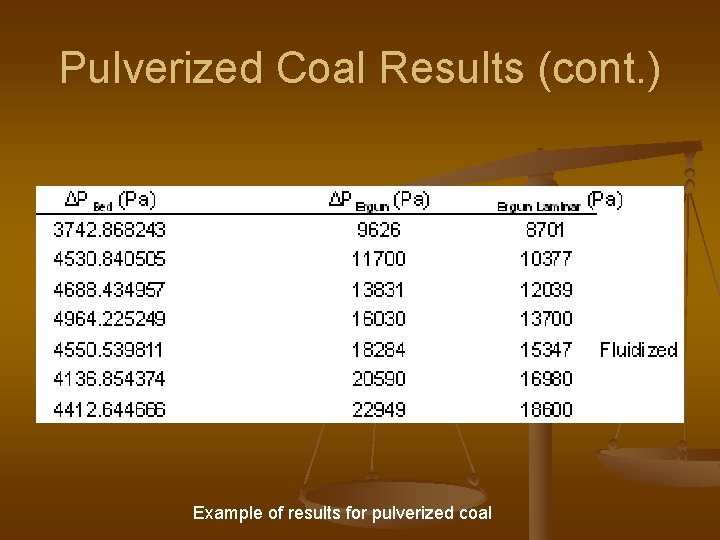Pulverized Coal Results (cont. ) Example of results for pulverized coalGlass Bead Results (cont. ) Figure 8: Pressure drop data and Ergun Equation for glass beads at 30. 0 °C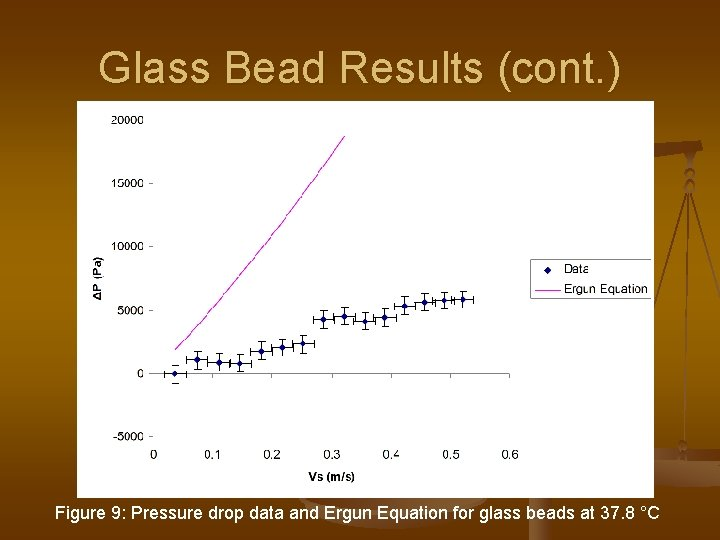Glass Bead Results (cont. ) Figure 9: Pressure drop data and Ergun Equation for glass beads at 37. 8 °C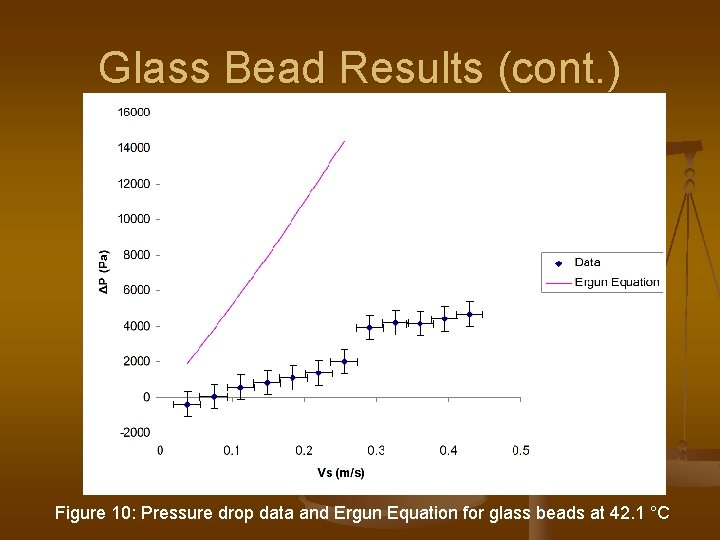Glass Bead Results (cont. ) Figure 10: Pressure drop data and Ergun Equation for glass beads at 42. 1 °C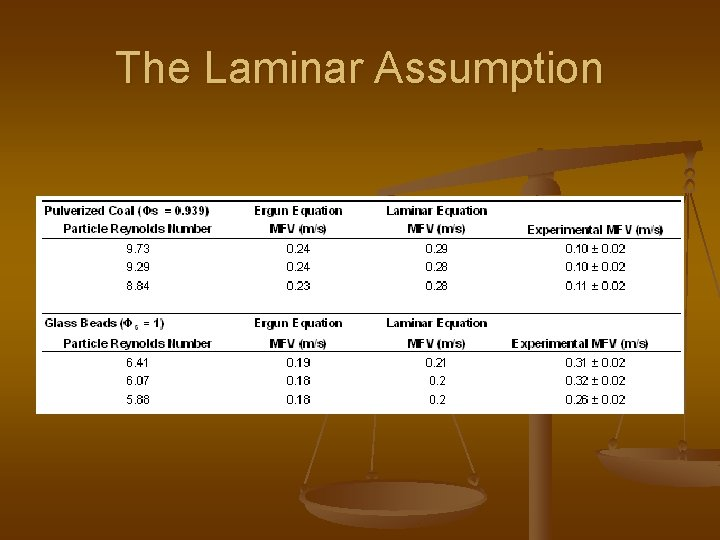The Laminar Assumption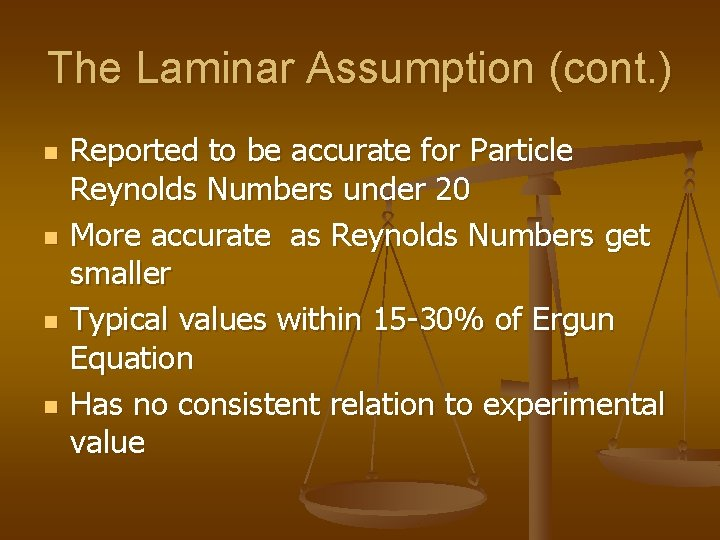The Laminar Assumption (cont. ) n n Reported to be accurate for Particle Reynolds Numbers under 20 More accurate as Reynolds Numbers get smaller Typical values within 15 -30% of Ergun Equation Has no consistent relation to experimental value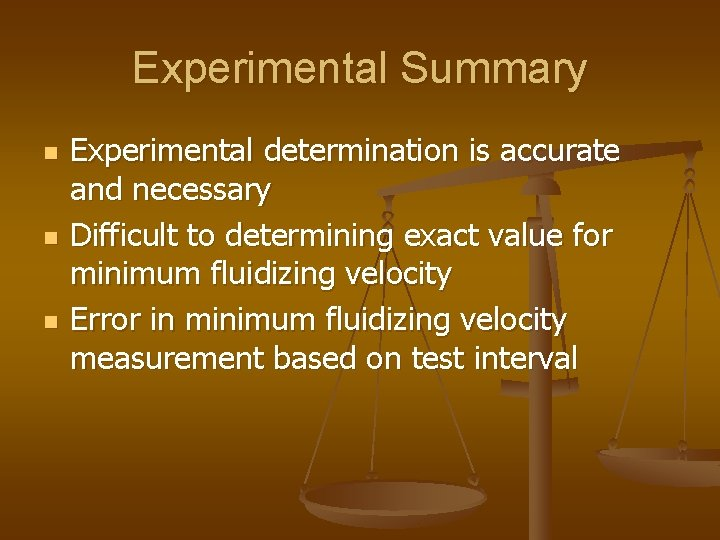Experimental Summary n n n Experimental determination is accurate and necessary Difficult to determining exact value for minimum fluidizing velocity Error in minimum fluidizing velocity measurement based on test interval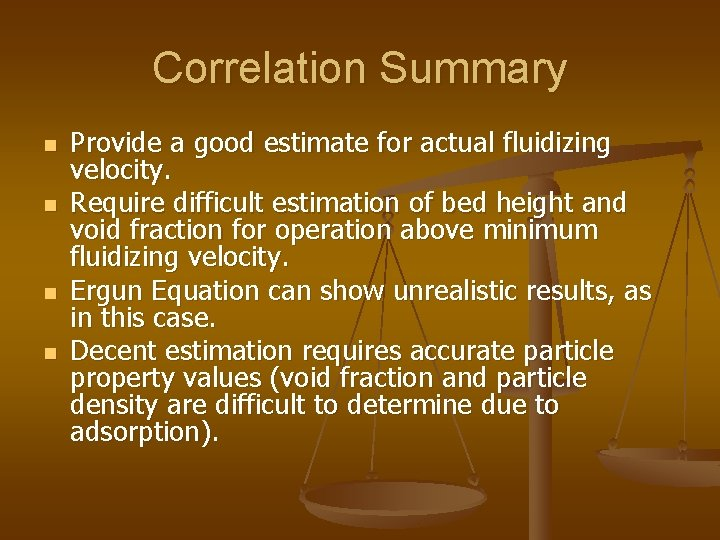Correlation Summary n n Provide a good estimate for actual fluidizing velocity. Require difficult estimation of bed height and void fraction for operation above minimum fluidizing velocity. Ergun Equation can show unrealistic results, as in this case. Decent estimation requires accurate particle property values (void fraction and particle density are difficult to determine due to adsorption).Conclusions n n n Correlations are useful, but not substitute for actual experimentation Experimentation necessary because of inaccurate and imprecise instrumentation Correlations are useful for industrial processes which are usually operated at two to three times the minimum fluidizing velocity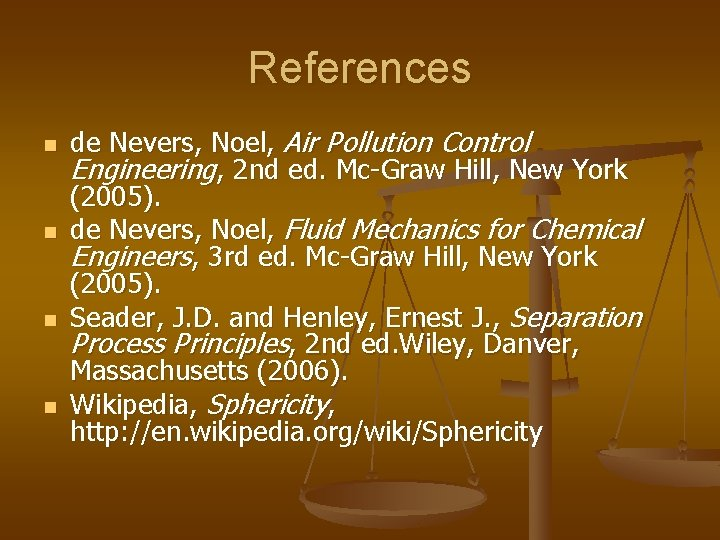References n n de Nevers, Noel, Air Pollution Control Engineering, 2 nd ed. Mc-Graw Hill, New York (2005). de Nevers, Noel, Fluid Mechanics for Chemical Engineers, 3 rd ed. Mc-Graw Hill, New York (2005). Seader, J. D. and Henley, Ernest J. , Separation Process Principles, 2 nd ed. Wiley, Danver, Massachusetts (2006). Wikipedia, Sphericity, http: //en. wikipedia. org/wiki/SphericityQuestions n 5 Minute Question Period